# The A.CRE Method for Doing Cap Rate Math in Your Head

In this post, I’d like to share a method that, with a little practice, will enable you to quickly do cap rate math in your head; whether it’s quickly figuring out what the sale or purchase price would be of a property based on the NOI and cap rate or, inversely, figuring out what the NOI should be based on a purchase or sale price and the cap rate.

This is a step-by-step guide on how this method works that includes study flash cards along the way so that you can test your understanding. I hope you find this both enjoyable and useful. Please feel free to reach out with any feedback as you try it out.

## Background to Developing this Method

The first step towards developing this method was to create a few tables with various NOIs, cap rates, and their resulting values; and to look for patterns that might be universal. When I created the table below, the first thing that was immediately apparent was the following: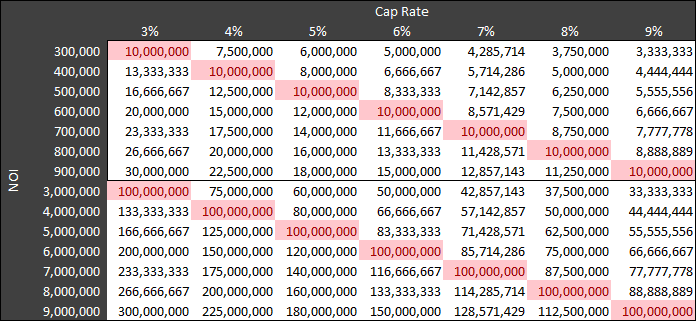All NOI numbers that began with X and were divided by an X% cap rate equaled a power of 10. More specifically, the formula would be the following:

X * 10y / (X/100) = 10y+2

NOTE: DO NOT WASTE ANY TIME TRYING TO MEMORIZE THIS FORMULA!

For example, a \$400k NOI with a 4% cap rate equals a \$10MM purchase price and a \$4MM NOI with a 4% cap rate equals \$100MM.

Soon after, I had another important realization:

If a \$400k NOI at a 4% cap rate was \$10MM, then every additional \$400k in NOI at a 4% cap rate would equal an additional \$10MM in value. So an \$800k NOI (\$400k x 2) at the same 4% cap rate would result in a \$20MM sale price (\$10MM * 2), or a \$1.2MM NOI (400k x 3) would be a \$30MM sale price.

This table below highlights this rule for different cap rates: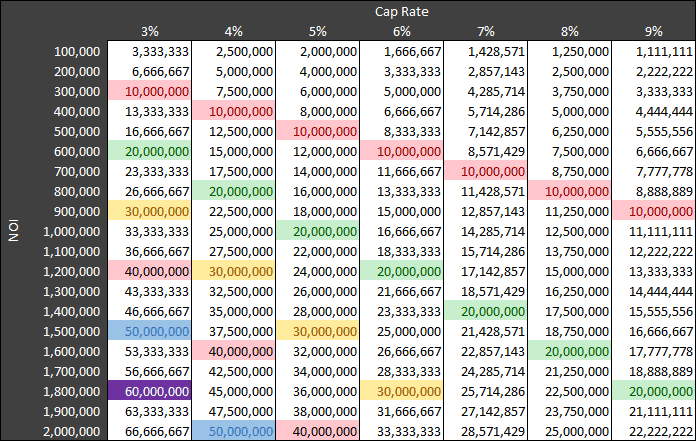While reading through the step-by-step guide below, keep the above in mind. By following the steps, working to memorize a series of fraction values as decimals, and some practice you should be able to derive rough property values based on cap rates quickly with nothing but a few seconds to think about it.

## The A.CRE Method to Doing Cap Rate Math in Your Head

#### Step 1:

Take the cap rate you are using and move the decimal point to the right until the cap rate number becomes a value that is as close as possible to the value of the NOI. For example, if the NOI is \$1,400,000 and your cap rate is 7%, or .07; then the value closest to the NOI based on moving the decimal point of the cap rate to the right would be 700,000. If you have an NOI of 3,000,000 and your cap rate is 4%, then the value closest to the NOI will be 4,000,000. The number derived from this first step will from hereon in be referred to as the Cap Rate Value Match (CRVM).

As with all the steps in the A.CRE method, this should be a fairly easy process. Try the 10 practice questions below. Use the arrows on the left and right to change questions.

##### Practice Flashcards

CRVM Practice

What is the CRVM?

Cap Rate: 5.0%

NOI: 4,100,000

Click to View the Question

.05 – Cap Rate

0.5

5

50

500

5,000

50,000

500,000

(4,100,000 – NOI)

5,000,000 – Value closest to NOI – CRVM

CRVM Practice
CRVM Practice

What is the CRVM?

Cap Rate: 6.9%

NOI: 450,000

Click to View the Question

.069 – Cap Rate

0.69

6.9

69

690

6,900

69,000

(450,000 – NOI)

690,000 – Value closest to NOI – CRVM

CRVM Practice
CRVM Practice

What is the CRVM?

Cap Rate: 8.4%

NOI: 700,000

Click to View the Question

.084 – Cap Rate

0.84

8.4

84

840

8,400

84,000

(700,000 – NOI)

840,000- Value closest to NOI – CRVM

CRVM Practice
CRVM Practice

What is the CRVM?

Cap Rate: 9.3%

NOI: 90,000

Click to View the Question

.093 – Cap Rate

0.93

9.3

93

930

9,300

(90,000 – NOI)

93,000 – Value closest to NOI – CRVM

CRVM Practice
CRVM Practice

What is the CRVM?

Cap Rate: 6.5%

NOI: 590,000

Click to View the Question

.065 – Cap Rate

0.65

6.5

65

650

6,500

65,000

(590,000 – NOI)

650,000 – Value closest to NOI – CRVM

6,500,000

CRVM Practice
CRVM Practice

What is the CRVM?

Cap Rate: 3.9%

NOI: 450,000

Click to View the Question

.039 – Cap Rate

0.39

3.9

39

390

3,900

39,000

390,000 – Value closest to NOI – CRVM

(450,000 – NOI)

3,900,000

CRVM Practice
CRVM Practice

What is the CRVM?

Cap Rate: 7.2%

NOI: 6,350,000

Click to View the Question

.072 – Cap Rate

0.72

7.2

72

720

7,200

72,000

720,000

(6,350,000 – NOI)

7,200,000 – Value closest to NOI – CRVM

CRVM Practice
CRVM Practice

What is the CRVM?

Cap Rate: 3.45%

NOI: 50,700,000

Click to View the Question

.0345 – Cap Rate

0.345

3.45

34.5

345

3,450

34,500

345,000

3,450,000

34,500,000 – Value closest to NOI – CRVM

(50,700,000 – NOI)

345,000,000

CRVM Practice
CRVM Practice

What is the CRVM?

Cap Rate: 9.0%

NOI: 9,700,000

Click to View the Question

.09 – Cap Rate

0.9

9

90

900

9,000

90,000

900,000

9,000,000 – Value closest to NOI – CRVM

(9,700,000 – NOI)

90,000,000

CRVM Practice
CRVM Practice

What is the CRVM?

Cap Rate: 4.7%

NOI: 16,500,000

Click to View the Question

.047 – Cap Rate

0.47

4.7

47

470

4,700

47,000

470,000

4,700,000 – Value closest to NOI – CRVM

(16,500,000 – NOI)

47,000,000

CRVM Practice

#### Step 2:

The second step is to take the CRVM from Step 1 and divide it by the cap rate to get what we will refer to from hereon as the Power of Ten Number (PTN). To do this is actually very easy. The PTN will always be a power of ten that is two digits larger than the CRVM (see the tables above in the ‘Background to Developing This Method’ section above for verification) . If the CRVM is 650,000, then dividing 650,000 by the 6.5% cap rate will give you 10,000,000 (the power of 10 that is two digits larger than 650,000). If the CRVM is 5,000,000, then dividing by 5% will give you a PTN of 100,000,000. In our two examples from Step 1, the PTN of a 700,000 CRVM is 10,000,000 and the PTN of a 3,000,000 CRVM is 100,000,000.

Before moving on to Step 3, let’s practice a few more examples utilzing both Step 1 and Step 2:

##### Practice Flashcards

PTN Practice

What is the PTN?

Cap Rate: 6.6%

NOI: 90,000

Click to View the Question

CRVM: 66,000

66,000/.066 = 1,000,000

or

The power of ten that is two digits greater than 66,000 = 1,000,000

PTN Practice
PTN Practice

What is the PTN?

Cap Rate: 3.33%

NOI: 90,000,127

Click to View the Question

CRVM: 33,300,000

33,300,000/.0333 = 1,000,000,000

or

The power of ten that is two digits greater than 33,300,000 = 1,000,000,000

PTN Practice
PTN Practice

What is the PTN?

Cap Rate: 7.0%

NOI: 9,127

Click to View the Question

CRVM: 7,000

7,000/.07 = 100,000

or

The power of ten that is two digits greater than 7,000 = 100,000

PTN Practice
PTN Practice

What is the PTN?

Cap Rate: 7.0%

NOI: 10,290,000

Click to View the Question

CRVM: 7,000,000

7,000,000/.07 = 100,000,000

or

The power of ten that is two digits greater than 7,000,000 = 100,000,000

PTN Practice
PTN Practice

What is the PTN?

Cap Rate: 4.3%

NOI: 1,000,000

Click to View the Question

CRVM: 430,000

430,000/.043 = 10,000,000

or

The power of ten that is two digits greater than 430,000 = 10,000,000

PTN Practice
PTN Practice

What is the PTN?

Cap Rate: 4.3%

NOI: 10,000,000

Click to View the Question

CRVM: 4,300,000

4,300,000/.043 = 100,000,000

or

The power of ten that is two digits greater than 4,300,000 = 100,000,000

PTN Practice
PTN Practice

What is the PTN?

Cap Rate: 3.9%

NOI: 850,000

Click to View the Question

CRVM: 390,000

390,000/.039 = 10,000,000

or

The power of ten that is two digits greater than 390,000 = 10,000,000

PTN Practice
PTN Practice

What is the PTN?

Cap Rate: 5.7%

NOI: 6,850,000

Click to View the Question

CRVM: 5,700,000

5,700,000/.057 = 100,000,000

or

The power of ten that is two digits greater than 5,700,000 = 100,000,000

PTN Practice
PTN Practice

What is the PTN?

Cap Rate: 5.4%

NOI: 60,000

Click to View the Question

CRVM: 54,000

54,000/.054 = 1,000,000

or

The power of ten that is two digits greater than 54,000 = 1,000,000

PTN Practice
PTN Practice

What is the PTN?

Cap Rate: 8.5%

NOI: 700,000

Click to View the Question

CRVM: 850,000

850,000/.085 = 10,000,000

or

The power of ten that is two digits greater than 850,000 = 10,000,000

PTN Practice

#### Step 3:

In Step 3, we figure out how many times, if at all, the CRVM can wholly fit into the NOI and take that number and multiply it by the PTN.

##### Example

Using the example of a \$1.4MM NOI and a 7% cap rate, the CRVM is \$700,000 and and can fit into the \$1,400,000 NOI 2 times. The PTN is 10,000,000 (700,000/.07 or the power of ten that is 2 digits larger than the CRVM). So, 2 (the amount of times the CRVM can fit completely into the NOI) times 10,000,000 (the PTN) equals \$20MM and thus, that is what you would pay for a property with a year 1 NOI of \$1.4MM and a cap rate of 7%.

There are still a couple more steps to learn and cap rates and NOI’s usually don’t fit so nicely together as they do in this example, but before we move on, let’s do some more practice examples that will give you the hang of this concept and then we can move forward to the next steps with more difficult examples.

Using CRVM & PTN to Derive Purchase Price

How much would you pay for this property?

NOI: 11,000,000

Cap Rate: 5.5%

Click to View the Question

CRVM: 5,500,000 (see step 1)

PTN: 100,000,000 (see step 2)

How many times does the CRVM fit into the NOI?
5,500,000 fits into 11,000,000 2 times.

2 x 100,000,000 = 200,000,000

Using CRVM & PTN to Derive Purchase Price
Using CRVM & PTN to Derive Purchase Price

How much would you pay for this property?

NOI: 1,050,000

Cap Rate: 3.5%

Click to View the Question

CRVM: 350,000 (see step 1)

PTN: 10,000,000 (see step 2)

How many times does the CRVM fit into the NOI?
350,000 fits into 1,050,000 3 times.

3 x 10,000,000 = 30,000,000

Using CRVM & PTN to Derive Purchase Price
Using CRVM & PTN to Derive Purchase Price

How much would you pay for this property?

NOI: 80,000

Cap Rate: 8%

Click to View the Question

CRVM: 80,000 (see step 1)

PTN: 1,000,000 (see step 2)

How many times does the CRVM fit into the NOI?
80,000 fits into 80,000 1 times.

1 x 1,000,000 = 1,000,000

Using CRVM & PTN to Derive Purchase Price
Using CRVM & PTN to Derive Purchase Price

How much would you pay for this property?

NOI: 950,000

Cap Rate: 4.75%

Click to View the Question

CRVM: 475,000 (see step 1)

PTN: 10,000,000 (see step 2)

How many times does the CRVM fit into the NOI?
475,000 fits into 950,000 2 times.

2 x 10,000,000 = 400,000,000

Using CRVM & PTN to Derive Purchase Price
Using CRVM & PTN to Derive Purchase Price

How much would you pay for this property?

NOI: 1,050,000

Cap Rate: 5.25%

Click to View the Question

CRVM: 525,000 (see step 1)

PTN: 10,000,000 (see step 2)

How many times does the CRVM fit into the NOI?
525,000 fits into 1,050,000 2 times.

2 x 10,000,000 = 20,000,000

Using CRVM & PTN to Derive Purchase Price
Using CRVM & PTN to Derive Purchase Price

How much would you pay for this property?

NOI: 24,000,000

Cap Rate: 6%

Click to View the Question

CRVM: 6,000,000 (see step 1)

PTN: 100,000,000 (see step 2)

How many times does the CRVM fit into the NOI?
6,000,000 fits into 24,000,000 4 times.

4 x 100,000,000 = 400,000,000

Using CRVM & PTN to Derive Purchase Price
Using CRVM & PTN to Derive Purchase Price

How much would you pay for this property?

NOI: 240,000

Cap Rate: 8%

Click to View the Question

CRVM: 80,000 (see step 1)

PTN: 1,000,000 (see step 2)

How many times does the CRVM fit into the NOI?
80,000 fits into 240,000 3 times.

3 x 1,000,000 = 3,000,000

Using CRVM & PTN to Derive Purchase Price
Using CRVM & PTN to Derive Purchase Price

How much would you pay for this property?

NOI: 15

Cap Rate: 5%

Click to View the Question

CRVM: 5 (see step 1)

PTN: 100 (see step 2)

How many times does the CRVM fit into the NOI?
5 fits into 15 3 times.

3 x 100 = 300

Using CRVM & PTN to Derive Purchase Price
Using CRVM & PTN to Derive Purchase Price

How much would you pay for this property?

NOI: 16,000,000

Cap Rate: 4%

Click to View the Question

CRVM: 4,000,000 (see step 1)

PTN: 100,000,000 (see step 2)

How many times does the CRVM fit into the NOI?
4,000,000 fits into 16,000,000 4 times.

4 x 100,000,000 = 400,000,000

Using CRVM & PTN to Derive Purchase Price
Using CRVM & PTN to Derive Purchase Price

How much would you pay for this property?

NOI: 1,800,000

Cap Rate: 6%

Click to View the Question

CRVM: 600,000 (see step 1)

PTN: 10,000,000 (see step 2)

How many times does the CRVM fit into the NOI?
600,000 fits into 1,800,000 3 times.

3 x 10,000,000 = 30,000,000

Using CRVM & PTN to Derive Purchase Price

##### For Steps 4 & 5, practice and Memorize These Fractions

Very rarely will we find cap rates and NOI’s set up so nicely for us as in the previous section. The reality is that we get more difficult variables like 1,550,000 at a 6% cap rate. As a result, in order to get good at this method of deriving approximate values from cap rates in your head, it is critical to memorize the following 35 fractions and their decimal equivalents by heart: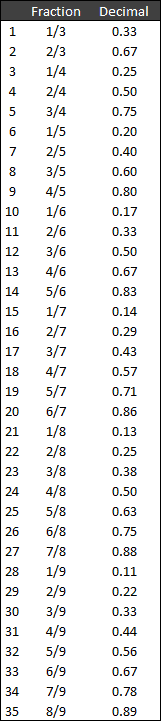##### Here are a few shortcuts, to help make this easier for you:

Fractions in this list that have the same decimal answer: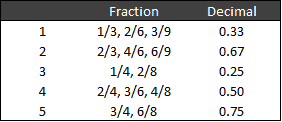Fractions with 9 in the denominator always have the numerator in perpetuity as the result: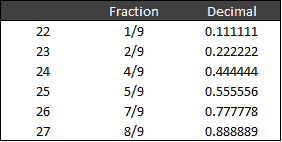Most of us can divide by 5 relatively easy: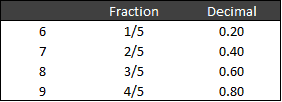And that leaves us with 12 fractions that many of us will have to commit to memory: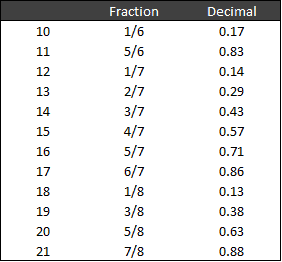##### Fraction Practice
Fractions to Decimals

1/4

2/8

Click to View the Question

0.25

Fractions to Decimals
Fractions to Decimals

2/9

Click to View the Question

0.222

Fractions to Decimals
Fractions to Decimals

5/7

Click to View the Question

0.71

Fractions to Decimals
Fractions to Decimals

1/3

2/6

3/9

Click to View the Question

0.33

Fractions to Decimals
Fractions to Decimals

1/5

Click to View the Question

0.20

Fractions to Decimals
Fractions to Decimals

1/7

Click to View the Question

0.14

Fractions to Decimals
Fractions to Decimals

2/7

Click to View the Question

0.29

Fractions to Decimals
Fractions to Decimals

3/4

6/8

Click to View the Question

0.75

Fractions to Decimals
Fractions to Decimals

3/7

Click to View the Question

0.43

Fractions to Decimals
Fractions to Decimals

4/5

Click to View the Question

0.80

Fractions to Decimals

##### Step 1-3 for a more challenging problem:

Before we get to Step 4, let’s first bring another example through Steps 1-3. Step 4 deals with valuing the part of the NOI that is left when you subtract the total CRVM’s that fit in the NOI from the NOI.

Example:

NOI: 1,650,000
Cap Rate: 6%

First, when doing mental math for cap rates, the idea is to get an approximate answer and it is not necessary to come up with the exact dollar, especially when having high level discussions. With this in mind, let’s round the NOI in this example to 1,700,000 and take it through Step 1. (If the NOI was in the hundred thousands, you should round to the nearest ten thousand)

Step 1: The CRVM here is \$600,000 –

.06 – Cap rate
0.6
6
60
600
6,000
60,000
600,000 – closest in value to to the NOI = CRVM
6,000,000

Step 2: Divide the CRVM by the cap rate to get a PTN of \$10,000,000 (600,000/.06)

Step 3: The CRVM fits in the NOI 2 times with \$500,000 left over. Taking the amount of times the CRVM fits in the NOI (2) and multiplying it by \$10,000,000 give us \$20,000,000.

So, \$1,200,000 (CRVM * 2) of the NOI at 6% is worth \$20,000,000

#### Step 4:

In step 4, we work in fractions and it will be the only part of this method that might be a bit challenging unless you have mastered the fraction conversions above.

The remaining value of the NOI that has yet to be valued, which in this example is \$500,000, will become the numerator and the CRVM, \$600,000, will become the denominator. The remaining value of the NOI and the CRVM will usually be easy to reduce to a simple fraction. In this case, 500,000/600,000 = 5/6. And if we have memorized our fractions above, we know that 5/6 equals 0.83. Since we rounded up our NOI pre-step 1, we should round down our decimal number to the nearest tenth to compensate. In this case, we can round down to 0.8

#### Step 5:

Multiply the PTN by 0.8. So 10,000,000 * 0.8 = \$8,000,000. Add that to the \$20,000,000 from step 3 and we have a value of ≈ \$28MM

The exact answer is \$27.5MM and \$28MM is only 1.8% above.

#### Wrap Up

That is all there is to it! As you get better with this, the goal is to just be on auto-pilot to the point where you are not thinking about the labels or steps and you just go through the process fluidly and automatically.  I hope the A.CRE Method for doing cap rate math in your head becomes a useful tool for you! Also, if any of our readers have any other tricks for doing cap rate math or any other back of the envelope math for real estate quickly in your head, I’d love to hear from you!

###### Practice Examples

Below are a couple practice examples. A few additional tips to help solve these problems:

• If you have an NOI that appears challenging, see if you can round it up or down to make it easier to work with. Just remember if you rounded the NOI up a significant amount, then the actual price will be a bit lower than your result, and vice versa if you rounded your NOI down.
• If you have a challenging cap rate, round it up or down to make it easier to work with. Like the NOI, remember if you are rounding your cap rate down significantly, your result will be higher and vice versa for rounding the cap rate up. A few basis points will not make much of a difference at all however.
Full Practice

NOI: 400,000

Cap Rate: 5%

Click to View the Question

\$8MM

CRVM: 500,000

PTN: 10,000,000

CRVM fits 0 times into the NOI

Remaining NOI to value: 400,000

400,000/500,000 (CRVM) = 4/5

4/5 = 0.8

PTN * 0.8 = 8,000,000

\$8MM

Full Practice
Full Practice

NOI: 1,350,000

Cap Rate: 5.5%

Click to View the Question

Approximately \$25MM

CRVM: 550,000

PTN: 10,000,000

CRVM fits 2 times into the NOI (1,100,000)

The purchase price of 1,100,000 = PTN * 2 (number of times the CRVM fits in the NOI) = 20,000,000

Remaining NOI to value: 250,000

250,000/550,000(CRVM) = approximately 1/2

1/2 = 0.50

PTN * 0.50 = 5,000,000

20,000,000 + \$5MM = approximately \$25MM

Full Practice
Full Practice

NOI: 2,451,327

Cap Rate: 7.5%

Click to View the Question

Approximately \$33MM

CRVM: 750,000

PTN: 10,000,000

CRVM fits 3 times into the NOI (2,250,000)

The purchase price of 2,250,000 = PTN * 3 (number of times the CRVM fits in the NOI) = 30,000,000

Remaining NOI to value: 251,327 (think approximately \$250k)

250,000/750,000 (CRVM) = approximately 2/7

2/7 = 0.29

PTN * 0.29 = 2,900,000

\$30MM + \$2.9MM = approximately \$32.9MM

Full Practice
Full Practice

NOI: 1,300,000

Cap Rate: 6%

Click to View the Question

Approximately \$22MM

CRVM: 600,000

PTN: 10,000,000

CRVM fits 2 times into the NOI (1,200,000)

The purchase price of 1,200,000 = PTN * 2 (number of times the CRVM fits in the NOI) = 20,000,000

Remaining NOI to value: 100,000

100,000/600,000 (CRVM) = approximately 1/6

1/6 = 0.167

PTN * 0.167 = 1,670,000

20,000,000 + \$1.67MM = approximately \$22MM

Full Practice
Full Practice

NOI: 940,000

Cap Rate: 5%

Click to View the Question

Approximately \$18MM

CRVM: 500,000

PTN: 10,000,000

CRVM fits 1 times into the NOI (500,000)

The purchase price of 500,000 = PTN * 1 (number of times the CRVM fits in the NOI) = 10,000,000

Remaining NOI to value: 440,000

440,000/500,000 (CRVM) = approximately 4/5

4/5 = 0.8

PTN * 0.8 = 8,000,000

\$10MM + \$8MM = approximately 18MM

Full Practice
Full Practice

NOI: 1,940,000

Cap Rate: 5%

Click to View the Question

Approximately \$38MM

CRVM: 500,000

PTN: 10,000,000

CRVM fits 3 times into the NOI (1,500,000)

The purchase price of 1,500,000 = PTN * 3 (number of times the CRVM fits in the NOI) = 30,000,000

Remaining NOI to value: 440,000

440,000/500,000 (CRVM) = approximately 4/5

4/5 = 0.8

PTN * 0.8 = 8,000,000

\$30MM + \$8MM = approximately 38MM

Full Practice
Full Practice

NOI: 695,000

Cap Rate: 6.63%

Alt:

Round cap rate

Round NOI

Click to View the Question

Approximately \$10MM

CRVM: 700,000 (round cap rate to 7%)

PTN: 10,000,000

CRVM fits 1 times into the NOI (700,000)

The purchase price of a \$700,000 NOI = PTN * 1 (number of times the CRVM fits in the NOI) = 10,000,000

Remaining NOI to value: 0 (round NOI up to 700k)

Approximately \$10MM

Full Practice
Full Practice

NOI: 3,327,000

Cap Rate: 4%

Hint: Round down and split NOI into two easy NOIs that will work well with 4%

Click to View the Question

Approximately \$83MM

The easiest way to do this would be to break the NOI down into 2 separate and simpler NOI’s – \$3MM & \$300k – and run the A.CRE method on each and add them back together because both fit very well with 4%.

CRVM: 4,000,000 & 400,000

PTN: 100,000,000 & 10,000,000

CRVM fits 0 times into the NOIs

Remaining NOI to value: 3,000,000 & 300,000

3,000,000/4,000,000(CRVM) & 300,000/400,000(CRVM) =  3/4

3/4 = 0.75

100,000,000 PTN * 0.75 = 75,000,000

10,000,000 PTN * 0.75 = 7,500,000

\$75MM + \$7.5MM = Approximately \$83MM

Full Practice
Full Practice

NOI: 695,000

Cap Rate: 6.15%

Alt:

Round cap rate down

Round NOI up

Click to View the Question

Approximately \$11MM

CRVM: 600,000 (round cap rate to 6.0%)

PTN: 10,000,000

CRVM fits 1 times into the NOI (600,000)

The purchase price of a 600,000 NOI = PTN * 1 (number of times the CRVM fits in the NOI) = 10,000,000

Remaining NOI to value: 100,000 (round NOI up to 700k)

100,000/600,000 (CRVM) = 1/6

1/6 = .17

PTN * 0.17 = 1,700,000

\$10MM + \$1.7MM = Approximately \$11MM

Since we rounded the cap rate down and the NOI up, we know our price is higher than what the actual price will be, so it makes sense to round down here.

Full Practice
Full Practice

NOI: 1,940,000

Cap Rate: 5%

Alt: solve by rounding the NOI up

Click to View the Question

A little less than \$38MM

Alternative Way

Round up NOI to \$2MM

CRVM: 500,000

PTN: 10,000,000

CRVM fits 4 times into the NOI (1,500,000)

The purchase price of 2,000,000 NOI = PTN * 4 (number of times the CRVM fits in the NOI) = 40,000,000

Remaining NOI to value: 0

Since you rounded the NOI up, in discussions you can say ‘a little less than \$40MM